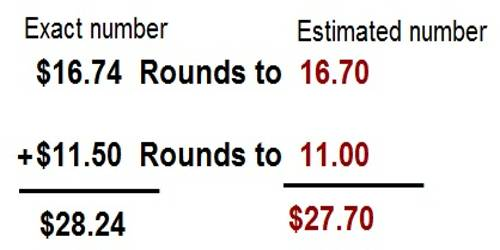Improving an Estimate of a sum by rounding

Estimation usually requires rounding. When you round a number, you find a new number that’s close to the original one. A rounded number uses zeros for some of the place values. If you round to the nearest ten, you will have a zero in the ones place. If you round to the nearest hundred, you will have zeros in the ones and tens places. Because these place values are zero, adding or subtracting is easier, so you can find an estimate to an exact answer quickly.A quick way to estimate the sum of two numbers is to round each number and then add the rounded numbers. This probably won’t be the exact answer but it may be close enough for some purposes.

How to Estimate a sum by rounding-

• Round each term that will be added
• Add the rounded numbers

An estimate can sometimes be improved. If the sum of 345 + 440 were estimated, we would round 345 to 300 and 440 to 400. The estimate would be 300 + 400 or 700. Both numbers were rounded down. The number 345 was rounded down by 45 and 440 was rounded down by 40. Adding 45 + 40 gives 85, which rounds to 100. Therefore, a better estimate would be 800. The actual sum is 785.

How to Improve the Estimate.

• Round each term that will be added
• Add the rounded numbers
• If both are rounded down or both rounded up see if the amount of rounding is more than 50. If it is, add or subtract 100 to the estimate.
• If one number is rounded down and the other is rounded up a closer estimate will not be produced by this method.

Some uses of rounding are:

• Checking to see if you have enough money to buy what you want.
• Getting a rough idea of the correct answer to a problem

Real life Example:

An estimate is an answer to a problem that is close to the solution, but not necessarily exact. Estimating can come in handy in a variety of situations, such as buying a computer. You may have to purchase numerous devices: a computer tower and keyboard for \$1,295, a monitor for \$679, the printer for \$486, the warranty for \$196, and software for \$374. Estimating can help you know about how much you’ll spend without actually adding those numbers exactly.

Information Source: